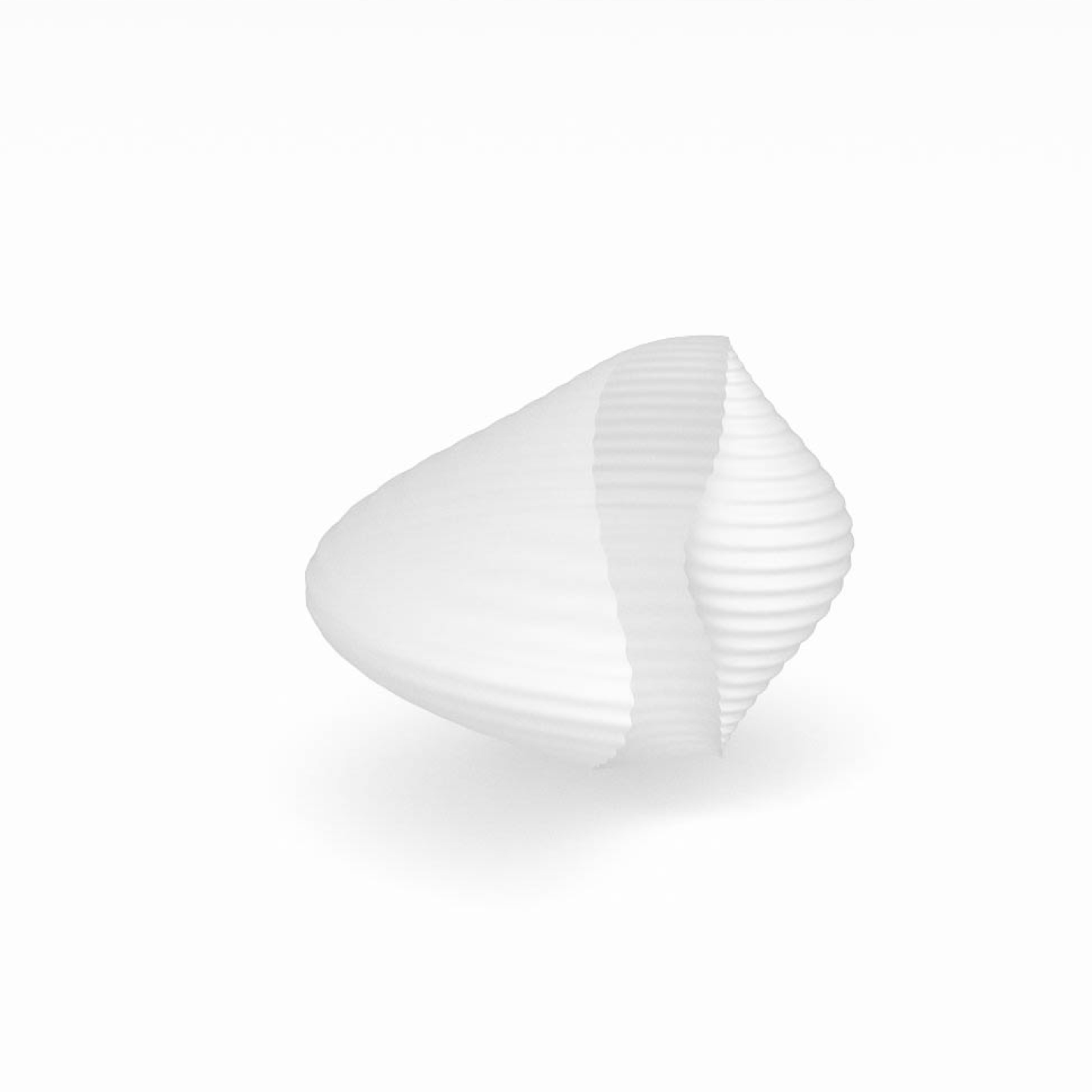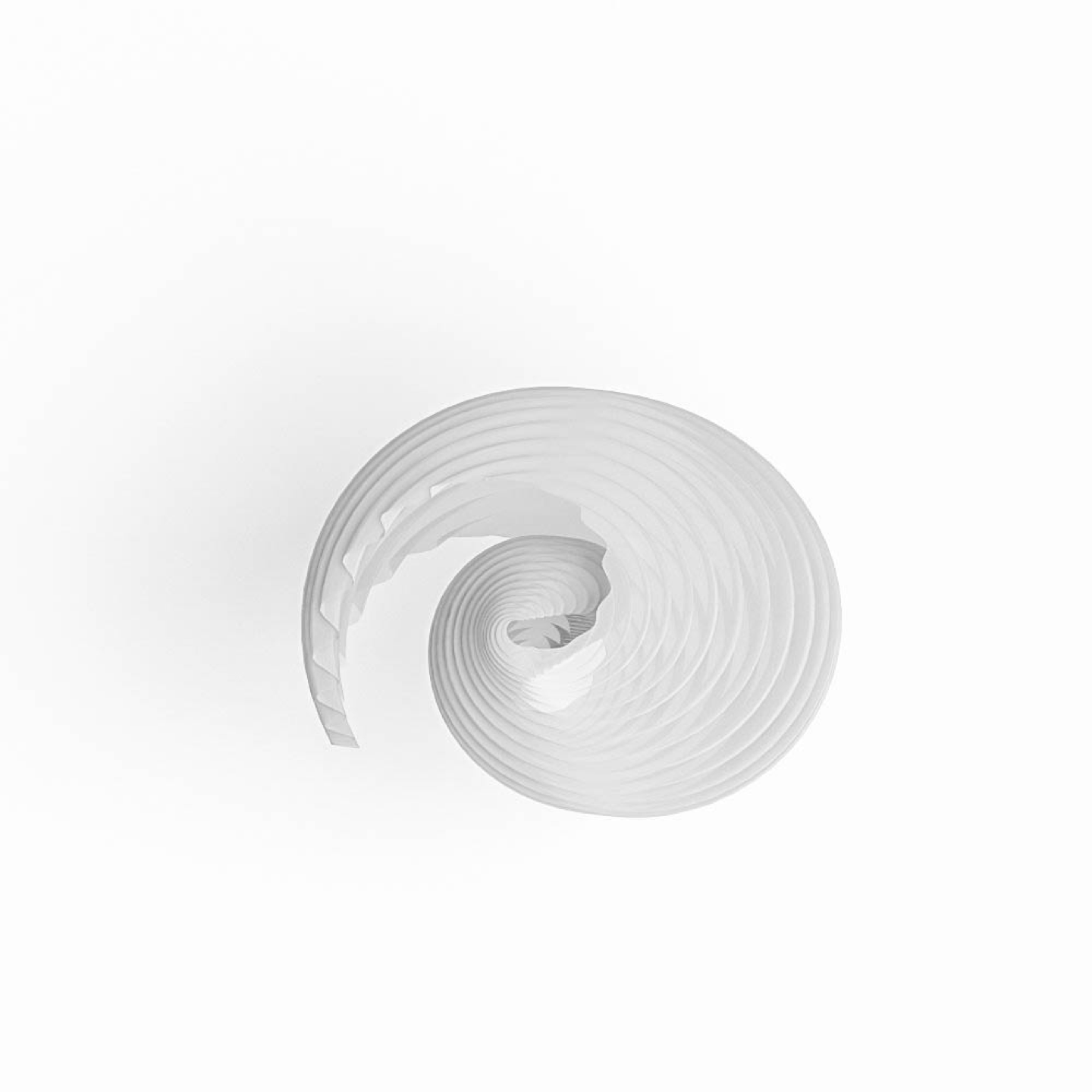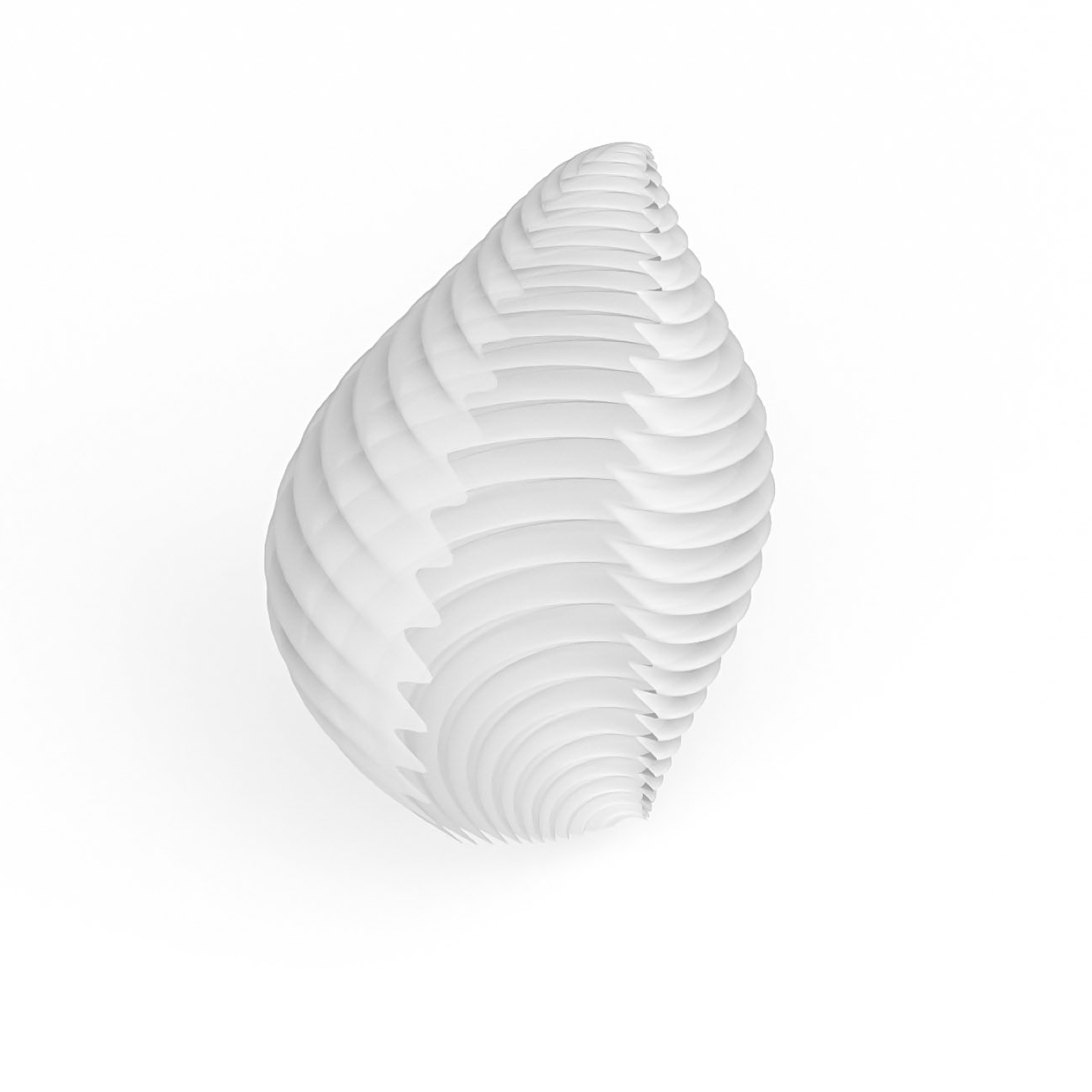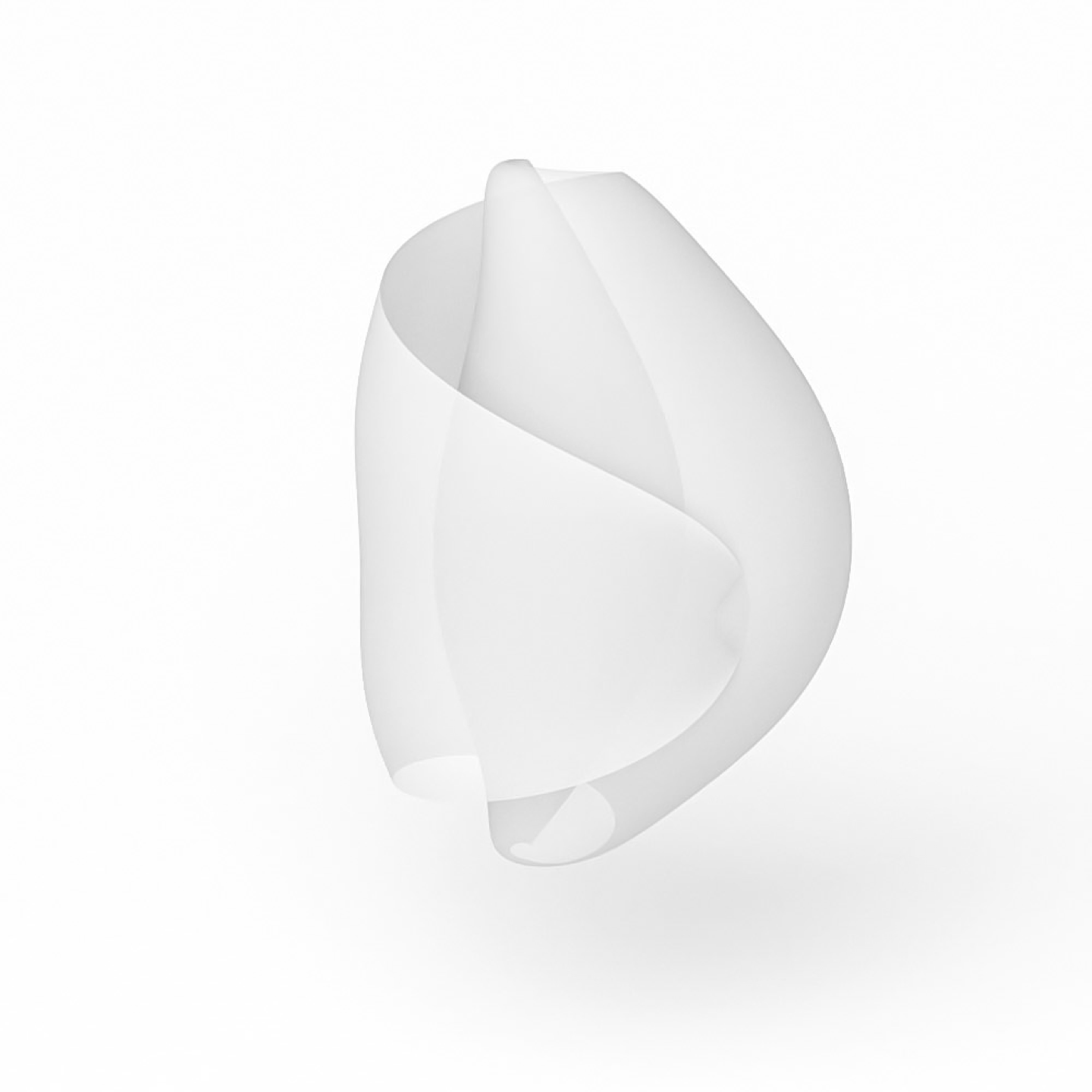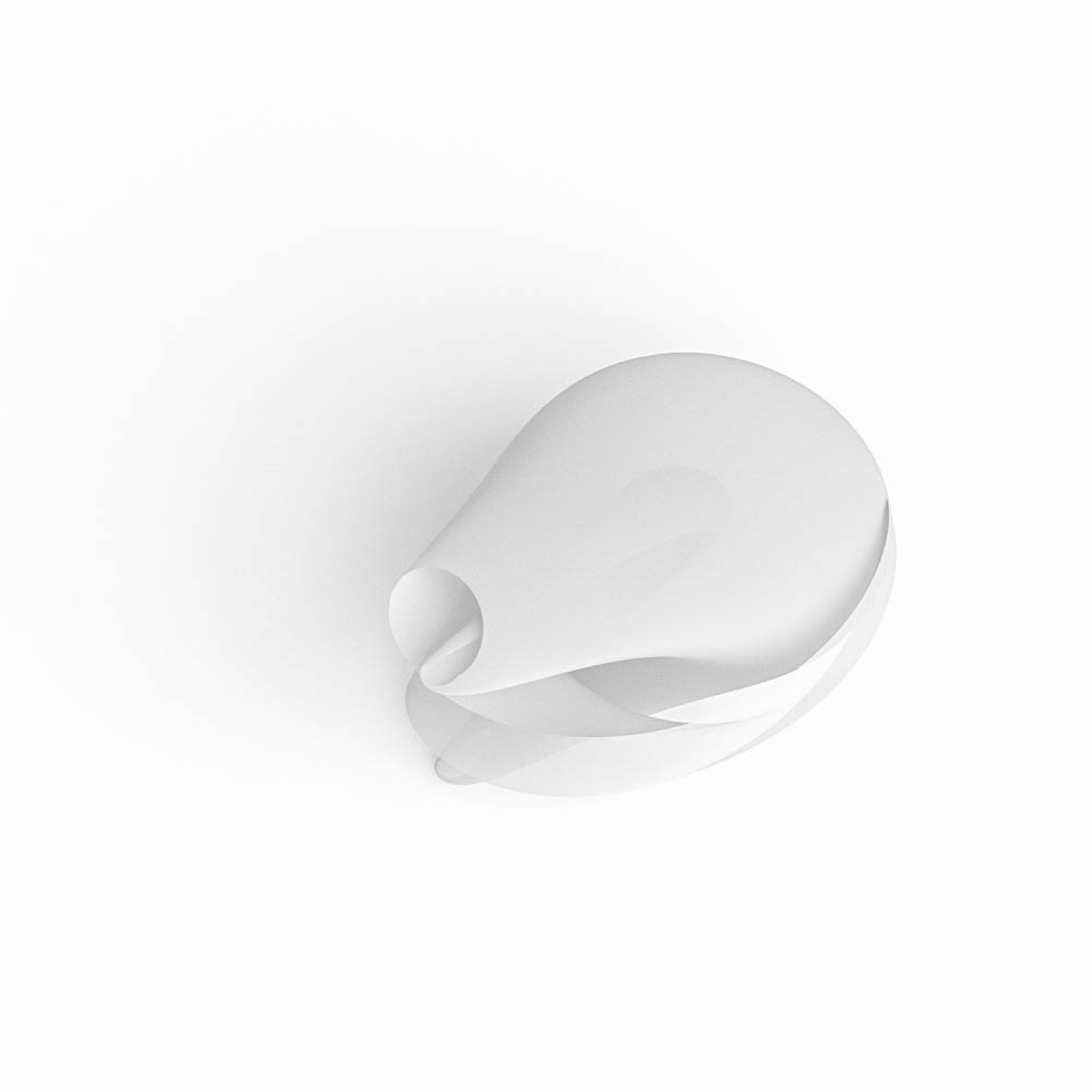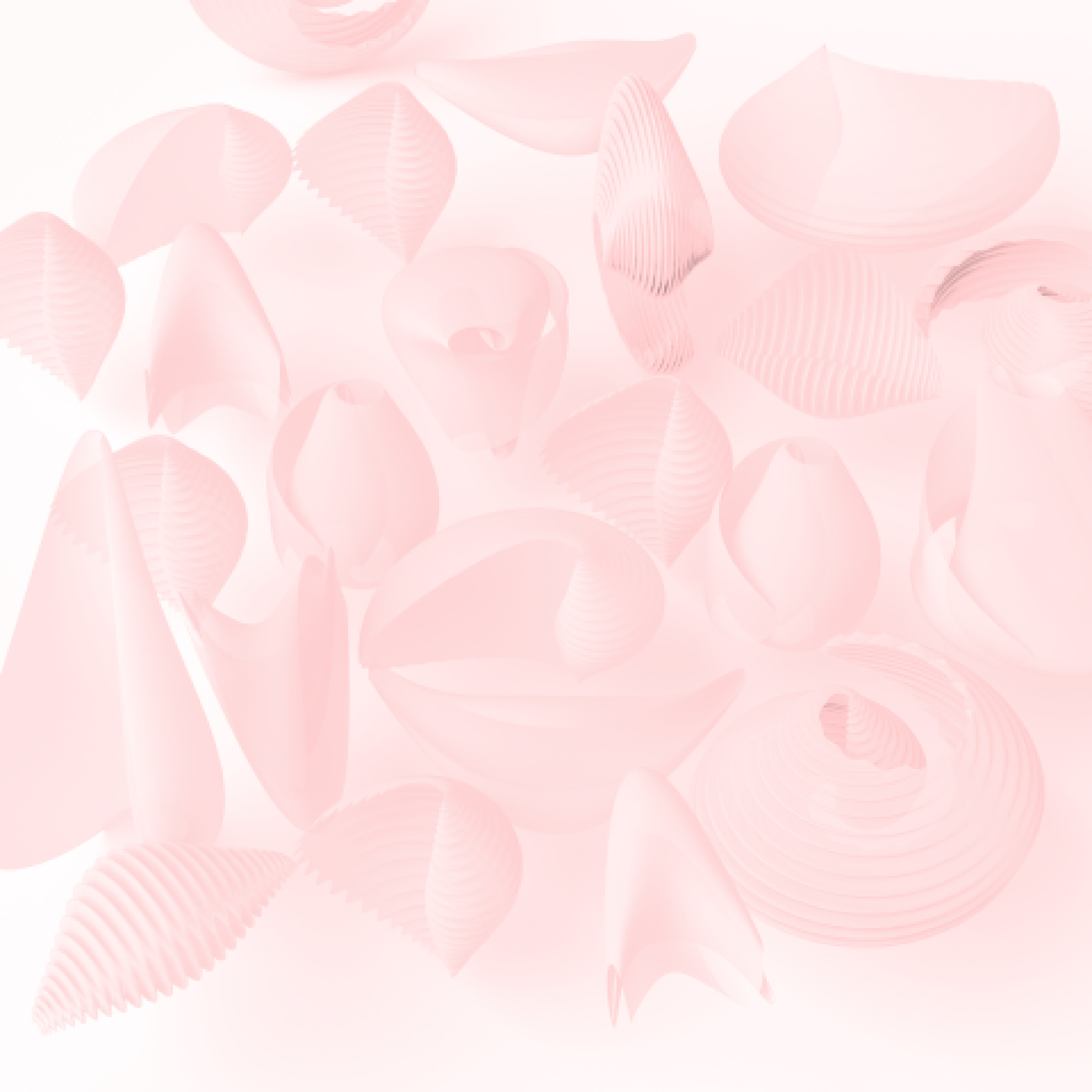# parametric pasta

With the help of George L. Legendre’s mathematical equations defining the shape of Italian pasta - conchiglie rigate, I created their 3D models.

Using the Python programming language inside the Grasshopper plug-in for Rhino 3D modeling software I added additional parameters to the form, like the intensity of grooving, what completely changes the appearance and creates fresh, unique and surprising shapes. Those can be easily adjusted playing with sliders for different parameters.

architectural studies m.sc.
modeling and programming course
homework assignment
timeline: 6 days

### equations of conchiglie rigate by george l. legendre### the code behind it

```import rhinoscriptsyntax as rs
from math​ import pi, cos, sin, pow

point_list = []
polygon_list = []

for i in range(0, spiral + 1):
for j in range(0, vertical + 1):
alfa = grooves * 0.25 * sin(j * pi / 250) * cos((6 * j + 25) * pi / 25)
beta = ((40 - i) / 40) * (0.3 + sin(j * pi / 250)) * pi
gamma = bend * 2.5 * cos(j / 125 * pi) + 2 * pow(sin((40 - i) / 80 * pi), 10) * pow(sin(j / 250 * pi), 10) * sin(j / 125 * pi + 1.5 * pi)
x = scale_xy * (alfa + cos(j / 125 * pi) + (5 + 30 * sin(j / 250 * pi)) * sin(beta) * sin(i / 40 * (0.1 * (1.1 + pow(sin(j / 250 * pi), 5))) * pi))
y = scale_xy * (alfa + (5 + 30 * sin(j / 250 * pi)) * cos(beta) * sin(i / 40 * (0.1 * (1.1 + pow(sin(j / 250 * pi), 5))) * pi) + gamma)
z = scale_z * 25 * cos((j / 250) * pi)
point_list.append([x, y, z])

for i in range(0, spiral):
for j in range(0, vertical):
p0 = i * (vertical + 1) + j
p1 = i * (vertical + 1) + j + 1
p2 = (i + 1) * (vertical + 1) + j + 1
p3 = (i + 1) * (vertical + 1) + j
polygon_list.append([p0, p1, p2, p3])

conchiglie = ghdoc.Objects.Geometries```
Using the Python programming language I created this component with sliders to change the different parameters.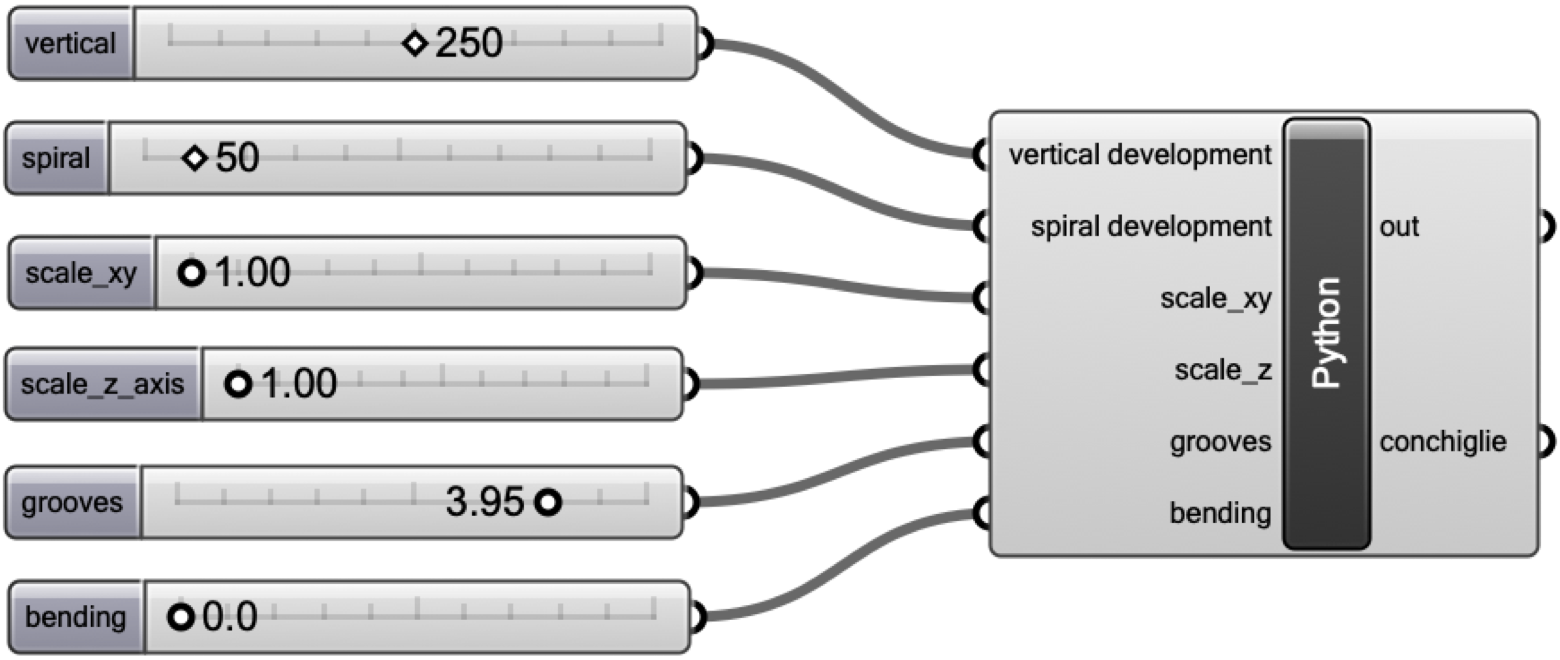The video below shows how the component works:

## setting parameters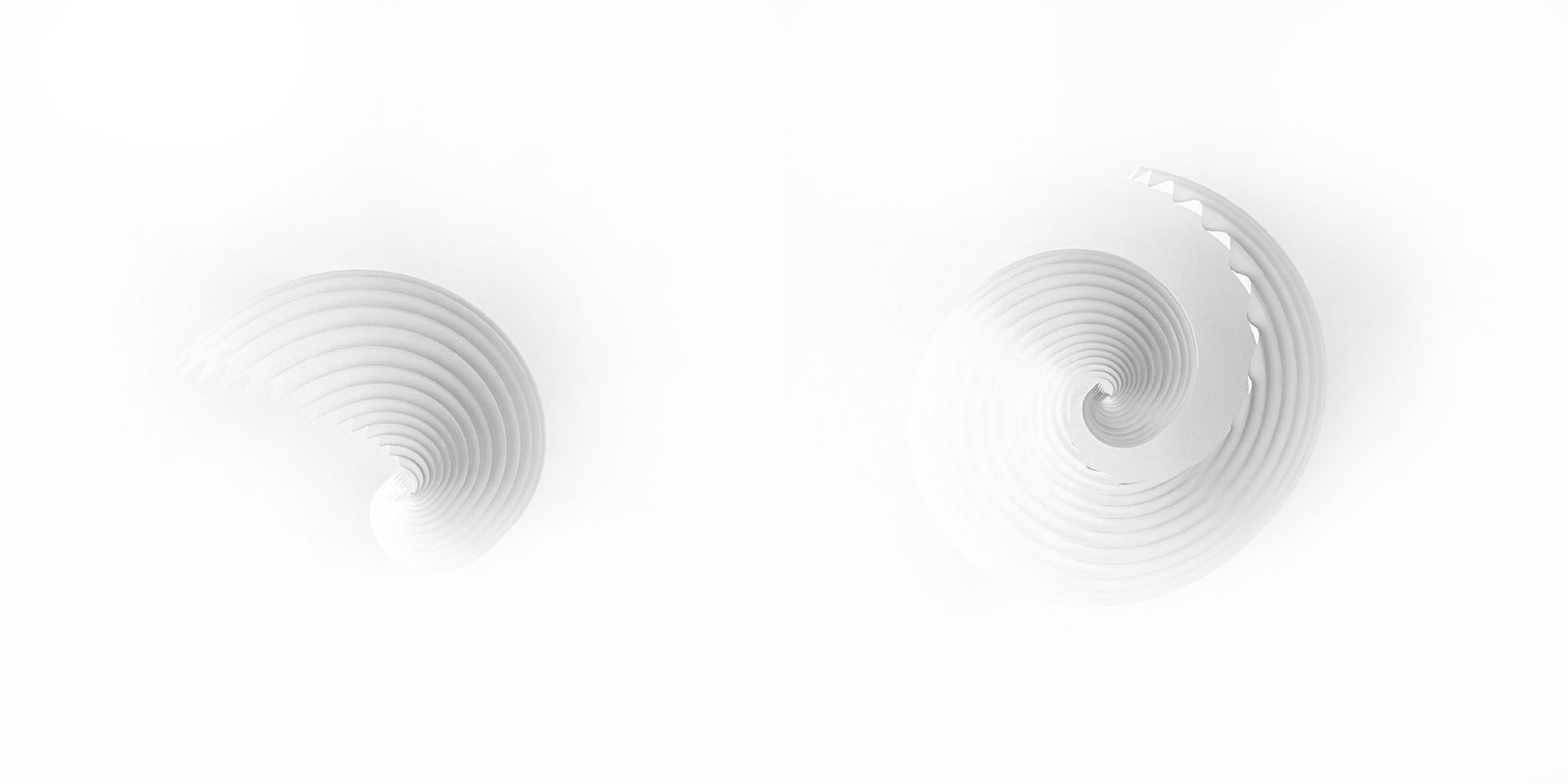spiral development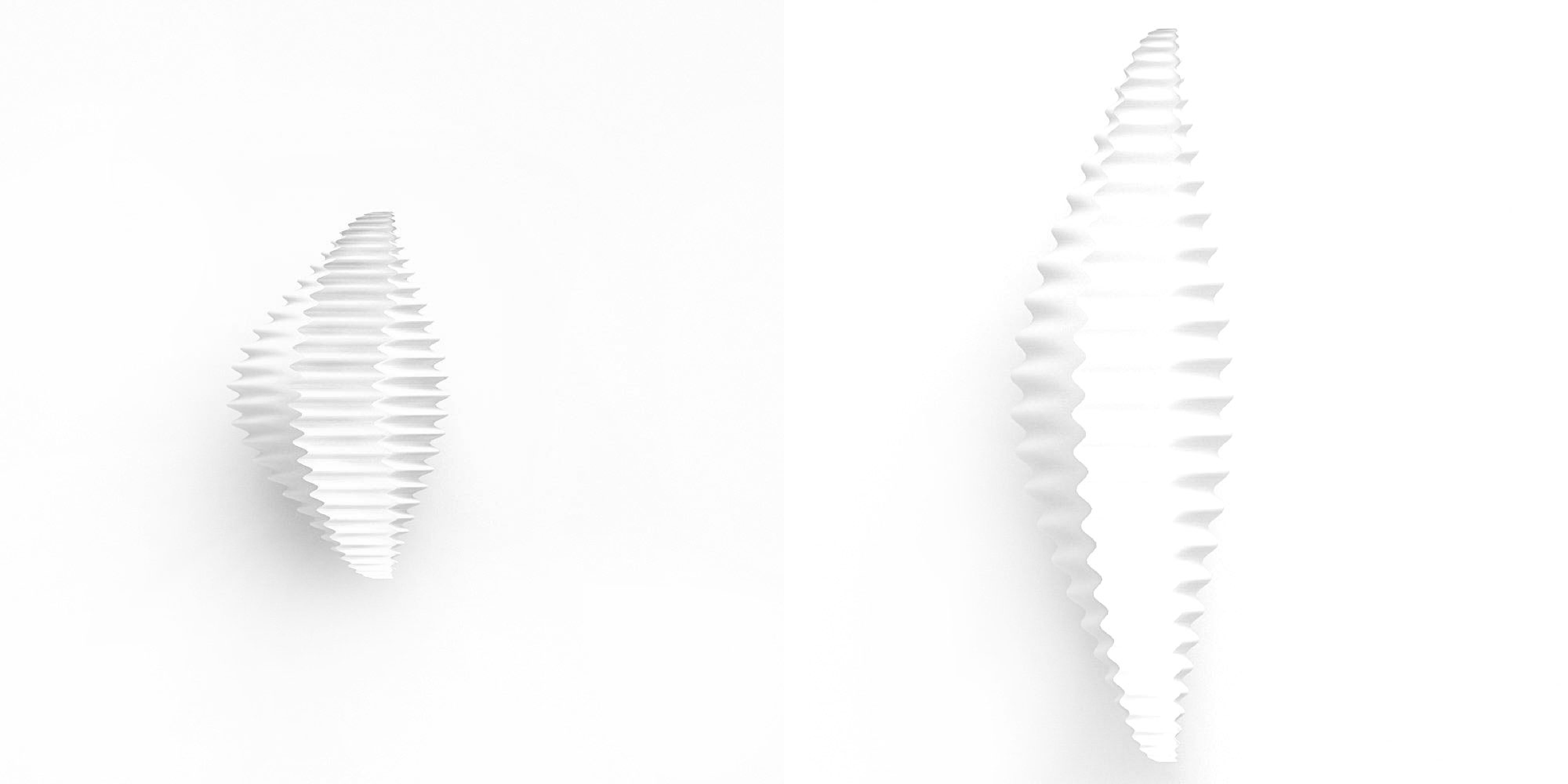vertical scaling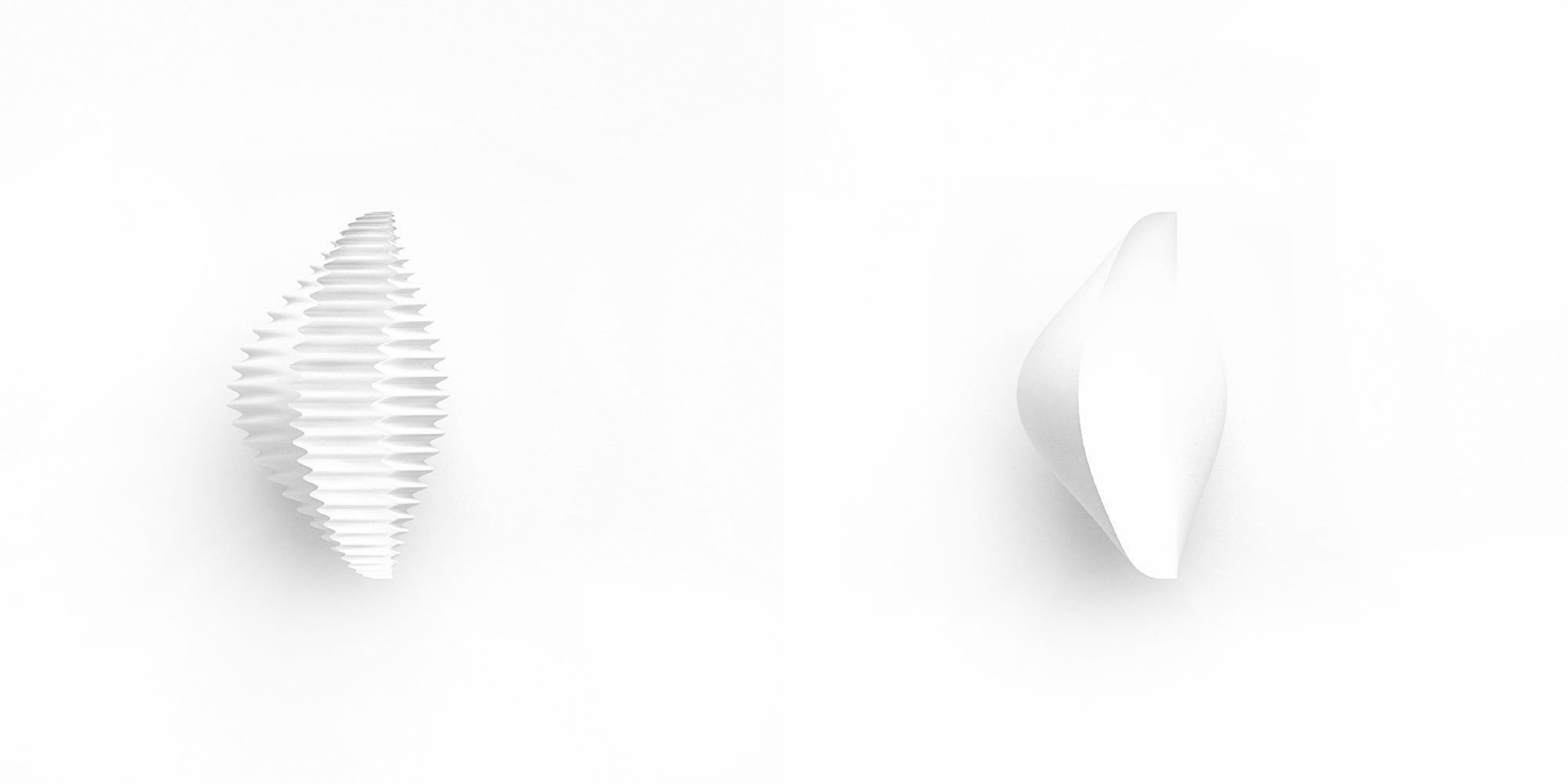grooves intensity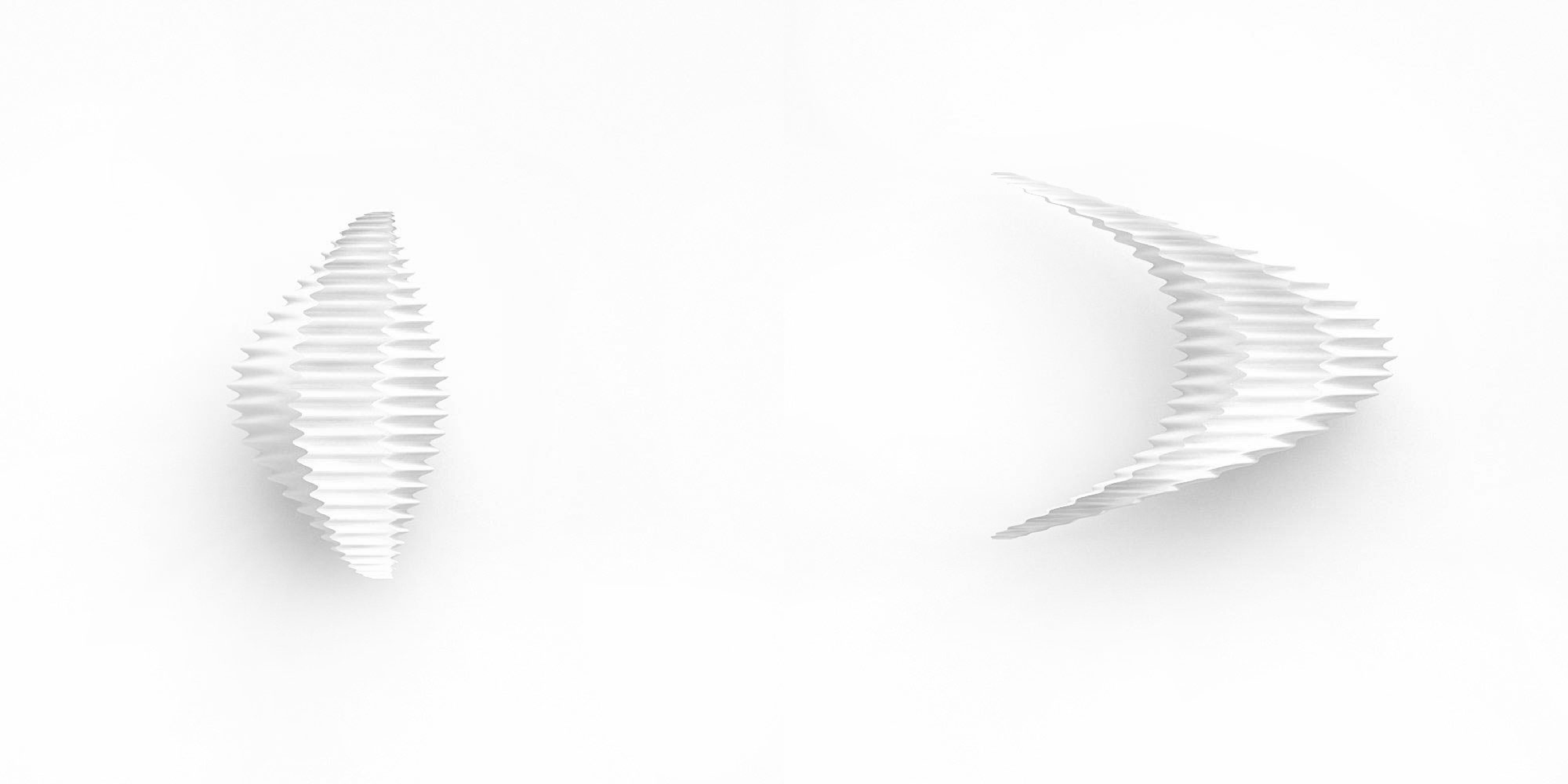bending

## outcomes with different parameters